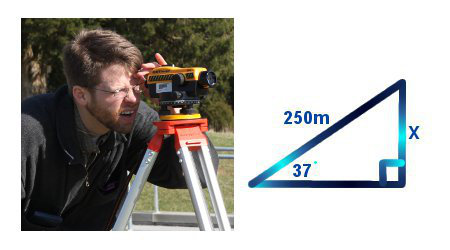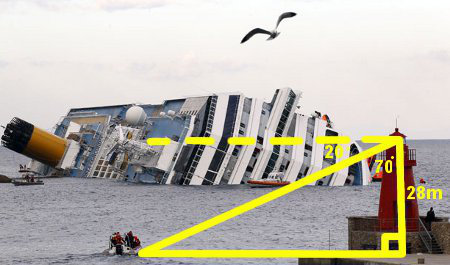# Trigonometry Angles Of Elevation And Depression

## Example One - Angle of Elevation

The Angle of Elevation is the angle between the horizontal up to the object.A surveyor standing at the base of a hill, uses a levelling device to see that the top of the hill is at an angle of elevation (angle between the horizontal up to the top of the hill) is 37°. She then walks up the slope of hill which she measures at 250 metres. What is the vertical height of the hill?

sin θ = O/H
sin 37 = x / 250
x = sin 37 × 250
x = 150.45

The vertical height of the hill is 150.45 metres.

## Did You Know That...?

Stremnaya Road in Bolivia is called the Death Road. An estimated 100 travellers each year die on this narrow winding mountain road with steep drops of 4000 metres.

## Example Two - Angle of Depression

The Angle of Depression is the angle between the horizontal down to the object.From the top of a lighthouse, a rescue coordinator can see a rescue boat at an angle of depression (angle between the horizontal down to the yacht) of 20°. He knows the lighthouse is 28 metres high. How far away from the base of the lighthouse is the boat?

If the angle of depression is 20 degrees, then the inside angle at the top of the triangle is 70 degrees.
tan θ = O/A
tan 70 = x / 28
x = tan 70 × 28
x = 76.93 metres

The rescue boat is 76.93 metres away from the base of the lighthouse.

## Question

The 10 metre long string attached to a kite is at an angle of elevation (angle between the horizontal ground up to the string) of 60°. How high is the kite?